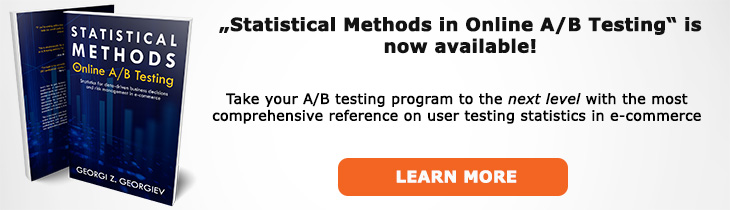# What does "Null Hypothesis" mean?

Definition of Null Hypothesis in the context of A/B testing (online controlled experiments).

## What is a Null Hypothesis?

The null hypothesis in the colloquial sense is a claim that we want to put to the test. It is usually the default, inherited state of matters, or a state of matters that would be preferred if no further information is available (we do not test). In other words, it should correspond to the position of a critic, skeptical to our proposed alternative or new treatment (the alternative hypothesis). In the strict statistical sense of the term it is defined more strictly as a statistical model (see hypothesis).

The null hypothesis is usually denoted by H0.

There are different kinds of null hypotheses, depending on the inference we want to make in the case at hand but in all cases the null and alternative should exhaust the entire parameter space (Θ0 ∪ Θ1 = Θ, Θ0 ∩ Θ1 = ∅). Most often in A/B tests we set up a superiority alternative such that the null hypothesis becomes H0: μ ≤ 0 or H0: μ ≤ m where m is some positive discrepancy. However, sometimes the alternative is that of non-inferiority so the null becomes H0 ≤ -m where m is the magnitude of the non-inferiority margin.

The null hypothesis should not be confused with the nil hypothesis (no difference hypothesis) although the two can coincide in the case of particular two-sided tests. The null hypothesis can be defined as any point or range which corresponds to a claim that needs to be tested, e.g. μ ≤ 2.

From a practical standpoint the null hypothesis is what gives the p-value a meaningful interpretation since the probability expressed by it is calculated under the assumption that the null is true. Upon observing a p-value below our chosen significance threshold we have sufficient evidence to reject the null hypothesis using the modus tollens logic or the argument from coincidence. We effectively shift the burden of proof to the one who would wand to make a claim corresponding to the null hypothesis.

The null can be redefined after a test is completed in order to check how warranted different conclusions are. For example, if you observed a given mean value x and the result is statistically significant for the original H0: μ < ≤ 0 if you were to define H10: μ ≤ x then you know immediately that you have poor evidence to reject H10 since the probability of observing this value assuming H10 is true is exactly 0.5, thus very likely.

A confidence interval can also be used to test a null hypothesis: every null hypothesis defined over any set of values not covered by the confidence interval can be rejected at significance level equal to 1 minus the confidence level, e.g. if a 95% confidence interval covers the values from 0.01 to +∞ then a null that covers the values from -∞ to 0.005 can be rejected at the 1-0.95 = 0.05 level.

## Articles on Null Hypothesis

Like this glossary entry? For an in-depth and comprehensive reading on A/B testing stats, check out the book "Statistical Methods in Online A/B Testing" by the author of this glossary, Georgi Georgiev.## Glossary Index by Letter

Select a letter to see all A/B testing terms starting with that letter or visit the Glossary homepage to see all.